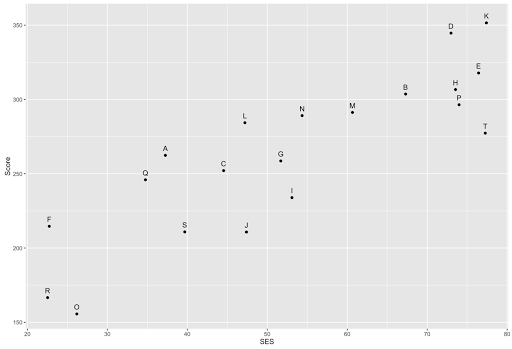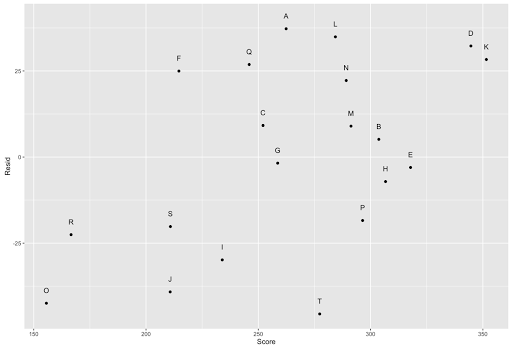[This article was first published on Data Literacy - The blog of Andrés Gutiérrez, and kindly contributed to R-bloggers]. (You can report issue about the content on this page here)
Want to share your content on R-bloggers? click here if you have a blog, or here if you don't.

In a previous entry, we talked about the meaning and importance of isolating confounding variables. This entry is dedicated to the residuals and its relation to the variable of interest when controlling for some confounding factors.

Let’s think about education. This example is always a good illustration to understand this issue. Assume that the performance of students on a standardized test is only related to Socio-Economic Status (SES) and the Ability of students. This way, consider the following model as a description of this matter:

$y = \beta_0 + \beta_1 \times SES + \beta_2 \times Ability + \varepsilon$

The previous model claims that a wealthy student tends to perform better in the test, besides a student with high ability will perform well. Note that Ability is a latent variable that is impossible to observe directly. However, if we control for SES, the residual term of the regression will represent the Ability of the student. This way, a model that we can fit in real life is given by:

$y = \beta_0 + \beta_1 \times SES + \epsilon = \hat{y} + \epsilon$

Note that the term $\hat{y}$ renders the performance of the student in the test explained by SES, while the term $\epsilon$ represents the performance of the student explained by unobservable variables such as Ability.

For instance, let’s assume that we collect data for 20 students including the performance in the test along with the SES. As the Ability is an unobservable variable, we only can adjust a model relating performance and SES. The following chart shows the ranking of students based on the test-score.This way, we can conclude that, from a test-based point of view, the student who performed better was Student K, and the one who performed worse were Student O. On the other hand, Student K is the wealthier one, and Students O is quite poor. This relation is very common in education: rich people perform better than poor people. However, when we explore the residuals’ behavior, we note that when plotting them against the Score, there is some linear trend that remains.That fact tells us two things: 1) the predicted values of the Score lacks the variable Ability and 2) The residuals do capture the behavior of that very variable. This way, if one would want to make a ranking (of the performance on the test) not based on SES but Ability, you must look to residuals.When deflacting by SES, things change. Now, the best one is not Student K (wealthier), but Students A (middle class). Besides that, the worst is not student O (poor), but Student T. However, we can claim that students K and O are not so far from the initial positions.

Here is the R code I applied to obtain the plots of this post.

rm(list = ls())

library(ggplot2)
set.seed(123)

N <- 20
ID <- LETTERS[1:N]
SES <- runif(N, 20, 80)
Ability <- runif(N, 0, 100)
Score <- 100 + 1 * Ability + 2 * SES + rnorm(N)

Schools <- data.frame(ID = ID, Score = Score,
SES = SES, Ability = Ability)

ggplot(Schools, aes(SES, Score, label = ID)) + geom_point() +
geom_text(vjust = 0, nudge_y = 3)

###########################
### Modelo Observable ###
###########################

fit <- lm(Score ~ SES, data = Schools)
Schools$Resid <- residuals(fit) # Los residuales están capturando el patrón de Ability ggplot(Schools, aes(Score, Resid, label = ID)) + geom_point() + geom_text(vjust = 0, nudge_y = 3) ggplot(Schools, aes(Ability, Resid, label = ID)) + geom_point() + geom_text(vjust = 0, nudge_y = 3) # Correlación bastante alta cor(Schools$Ability, Schools\$Resid)